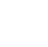Back to Chapter
Getting StartedBasic Syntax & DeclarationControl StatementsNo items found.
Function & EventsNo items found.
Array and its TypesNo items found.
OOP : Object Oriented ProgrammingNo items found.
Javascript StandardsNo items found.
HTML DOMNo items found.No items found.No items found.No items found.

# Question

Create a Program to find the factorial of a number using recursion.

# Hint

Take a number input from the user By using Prompt.

Now create a function

Inside Function, check if the number is greater than 0 then use recursion (number - 1)

Return the Final Value after the multiplying all outputs.

# Solution

``` function myFactorial(x) { if (x == 0) { return 1; } else { return x * myFactorial(x - 1); } } var num = prompt('Enter a positive number: '); if (num >= 0) { var result = myFactorial(num); console.log("The factorial of "+ num + " is " + result); }else { console.log('Enter a positive number.'); } ```